# Welcome

Hello! I thought it’d be interesting to benchmark an optimizer on:

• Constant LR vs. OneCycle LR Policy
• Between training sessions: Making a new optimizer vs. Preserve state by using the same optimizer

I’m curious to see if maintaining an optimizer state between trainning sessions can impact a model’s performance. In PyTorch, optimizers hold both a `state` and `param_groups`.

• `state` refers to a set of variables that are changed periodically by stepping with the optimizer, such as momentum’s accumlating gradients, or parameter-based learning rates modifiers.
• `param_groups` refers to the set of hyperparameters that are set upon optimizer initialization or changed through iterative use of an `lr_scheduler`, such as the `lr`, `beta`, `eps`, or `weight_decay`.

All of the below figures and code snipets can be found in the GitHub Repo. (Figures use Tensorboard, all code lies within Jupyter Notebooks)

## Benchmark Layout: Model, Datasets, and Task

Model: ResNet50 (Not pretrained, architecture from torchvision model zoo

Datasets:

• Imagenette
• Imagewoof

These are subsets of imagenet. See this repo for more information. In a nut shell, these datasets are great to run quick experiments with. Imagenette contains 10 easy classes from Imagenet, while Imagewoof contains 10 harder classes from Imagenet.

Imagenette classes: tench, English springer, cassette player, chain saw, church, French horn, garbage truck, gas pump, golf ball, parachute

Imagewoof classses: 10 different dog breeds, hence the name Imagewoof

Very Important Note:

‘Imagenette’ is pronounced just like ‘Imagenet’, except with a corny inauthentic French accent. If you’ve seen Peter Sellars in The Pink Panther, then think something like that. It’s important to ham up the accent as much as possible, otherwise people might not be sure whether you’re refering to “Imagenette” or “Imagenet”.

## A Quick Optimizer Review

What’s in a optimizer?

``````model = torchvision.models.resnet50(pretrained=False)

opt
``````
``````Output:
Parameter Group 0
betas: (0.9, 0.999)
eps: 1e-08
lr: 0.001
weight_decay: 0
)
``````

PyTorch optimizer’s contain a `state_dict`:

``````opt.state_dict().keys()
``````
``````Output:
dict_keys(['state', 'param_groups'])
``````

An optimizer’s `state_dict` contains two keys `state` and `param_groups`.

Currently, our `state` is empty since we haven’t trained/stepped with our optimizer:

``````opt.state_dict()['state']
``````
``````Output:
{}
``````

Currently, our `param_groups` have the following hyperparmeters:

``````opt.state_dict()['param_groups']
``````
``````Output:
{[{'lr': 0.001,
'betas': (0.9, 0.999),
'eps': 1e-08,
'weight_decay': 0,
'params': [140328111909616,
140328113037216,
140328116557456,
...]}
``````

Note that since we’re using the state_dict, we can only view this information, but not edit/modify it. `state_dicts` are imutable, unless you manually craft your own and call `opt.load_state_dict(some_dict)`.

A more common way to change a hyperparameter in a param_group is to use `opt.param_groups` rather than playing with `state_dicts`:

``````opt.param_groups.keys()
``````
``````Output:
dict_keys(['params', 'lr', 'betas', 'eps', 'weight_decay', 'amsgrad'])
``````

``````opt.param_groups['lr']
``````
``````Output:
0.001
``````

``````opt.param_groups['lr'] = 5e-2
opt.param_groups['lr']
``````
``````Output:
0.05
``````

``````opt.state_dict()['param_groups']
``````
``````Output:
[{'lr': 0.05,
'betas': (0.9, 0.999),
'eps': 1e-08,
'weight_decay': 0,
'params': [140328111909616,
140328113037216,
140328116557456,
...]}
``````

This means if you want to change one of the hyperparameters of your optimizer, you have one of two options:

1. Change the hyperparameter using the `param_groups`, which will preserve `state`
``````opt.param_groups['lr'] = 5e-2
opt.param_groups['lr']
``````
2. Make a fresh new `opt`
``````opt = torch.optim.Adam(model.parameters(), lr=5e-2)
``````

## A Quick LR Scheduler Review

Rather than manually changing the `lr` during training, we can use PyTorch’s `lr_schedulers`. An example of creating a OneCycleLR Schedule is below:

``````model = torchvision.models.resnet50(pretrained=False)
lr_scheduler = torch.optim.lr_scheduler.OneCycleLR(opt, max_lr=1e-3, epochs=5, steps_per_epoch=100)

opt
``````
``````Output:
Parameter Group 0
base_momentum: 0.85
betas: (0.95, 0.999)
eps: 1e-08
initial_lr: 4e-05
lr: 3.9999999999999996e-05
max_lr: 0.001
max_momentum: 0.95
min_lr: 4e-09
weight_decay: 0
)
``````

Note the difference between printing out the base `opt` in the previous section versus the lr_scheudler wrapped `opt`. This new opt contains more hyperparameter settings. These additional settings are used to adjust the base `opt` original hyperparameters as we step with the `lr_scheduler`. The OneCycle LR Scheduler needs to know the total number of steps beforehand, in order to adjust the `lr` appropriately between `min_lr` and `max_lr`.

To use the OneCycle `lr_scheduler`, we need to step with our `lr_scheduler` everytime with step with our `opt` in our training loop. An example of how the `lr` changes with respect to number of steps can be seen below.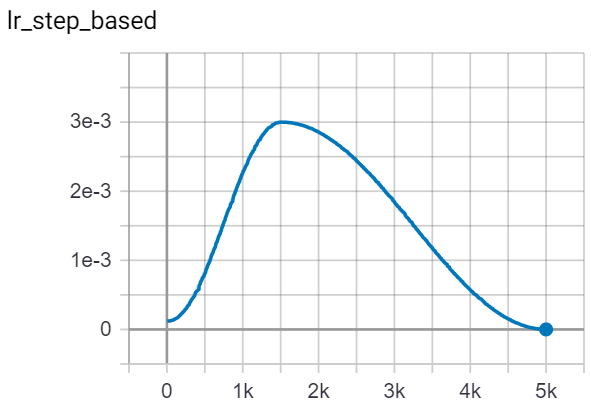To start another training session with the same optimizer, we can:

``````# Manually change LR
opt.param_groups['initial_lr'] = 5e-2
opt
``````
``````Output:
Parameter Group 0
base_momentum: 0.85
betas: (0.95, 0.999)
eps: 1e-08
initial_lr: 0.05
lr: 3.9999999999999996e-05
max_lr: 0.001
max_momentum: 0.95
min_lr: 4e-09
weight_decay: 0
)
``````

``````# Re-wrap the opt
lr_scheduler = torch.optim.lr_scheduler.OneCycleLR(opt, max_lr=1e-1, epochs=5, steps_per_epoch=100)

opt
``````
``````Output:
Parameter Group 0
base_momentum: 0.85
betas: (0.95, 0.999)
eps: 1e-08
initial_lr: 0.05
lr: 0.05
max_lr: 0.1
max_momentum: 0.95
min_lr: 4e-07
weight_decay: 0
)
``````

This means if you want to make a `lr_scheduler` with specific hyperparameter settings, you have two options:

1. Wrap an existing `opt` but first modify `param_groups`, which will preserve `state`
``````opt.param_groups['initial_lr'] = 5e-2
lr_scheduler = torch.optim.lr_scheduler.OneCycleLR(opt, max_lr=1e-1, epochs=5, steps_per_epoch=100)
``````
2. Wrap a fresh new `opt`
``````model = torchvision.models.resnet50(pretrained=False)
lr_scheduler = torch.optim.lr_scheduler.OneCycleLR(opt, max_lr=1e-3, epochs=5, steps_per_epoch=100)
``````

## The Experiment

Question: How impactful is the `state` in an optimizer, is it ok to throw it away between training sessions?

Experiment: Train a model for multiple training sessions, and benchmark the loss and classification accuracy with respect to the method of creating/modifying optimizers in between each training session.

An example training pipeline:

1. Make a model
2. Make an opt/lr_scheduler for the first time.
3. Train for 10 epochs
4. opt/lr_scheduler method
5. Train for 10 epochs
6. opt/lr_scheduler method
7. Train for 5 epochs

Where each opt/lr_scheduler method refers to the following techniques to create/modify optimizers:

1. Make a new optimizer from initialization, `torch.optim.Adam()`
2. Modify an existing optimizer to preserve `state` by modifying `param_groups`
3. Wrap a `lr_scheduler` on a new optimizer, `torch.optim.Adam()`
4. Wrap a `lr_scheduler` an existing optmizier to preserve `state` by modifying `param_groups`

For this experiment, I used the `Adam` optimizer and `OneCycleLR` LR Scheduler

Total Models Trained: 2 Datasets, 4 methods = 8 models.

## Results - Imagenette

Below is a set of figures corresponding to Training Loss, Training Accuracy, Validation Loss, Validation Accuracy for the Imagenette Dataset.

Legend: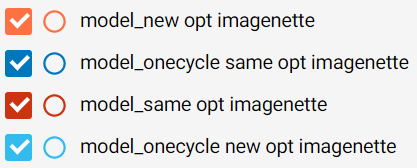Figures:

Train Validation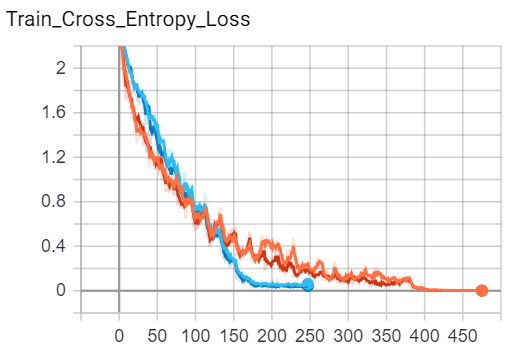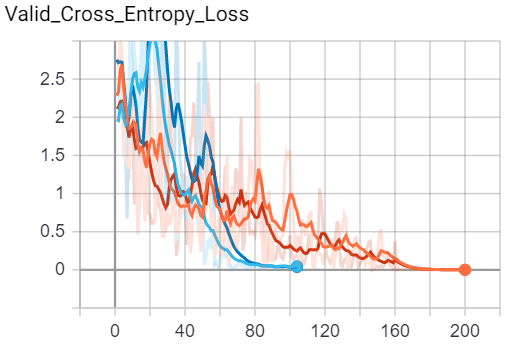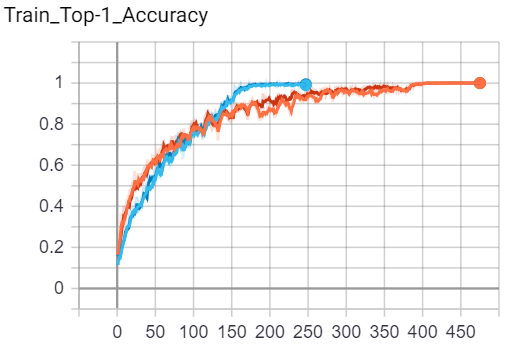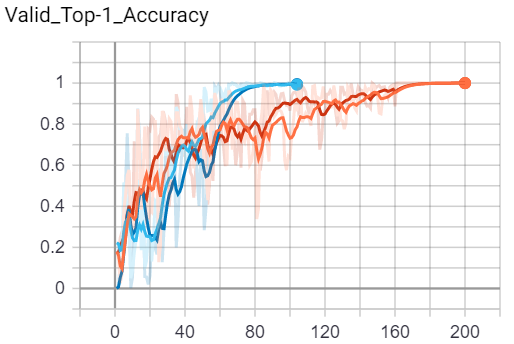## Results - Imagewoof

Below is a set of figures corresponding to Training Loss, Training Accuracy, Validation Loss, Validation Accuracy for the Imagewoof Dataset.

Legend: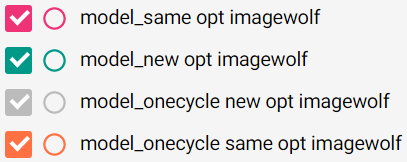Figures:

Train Validation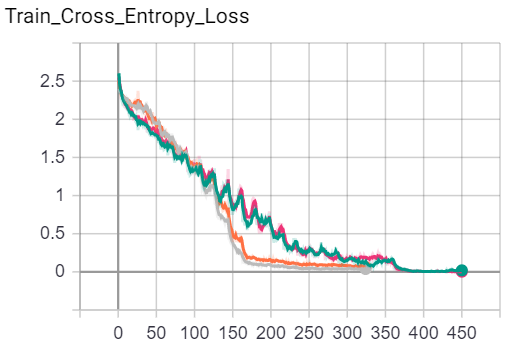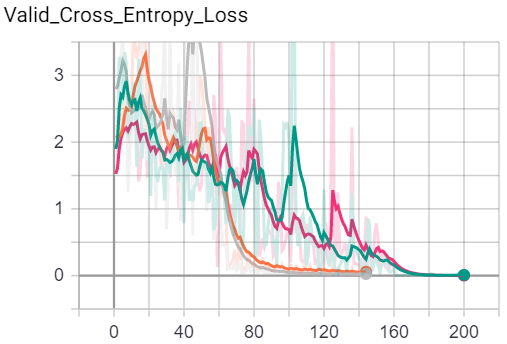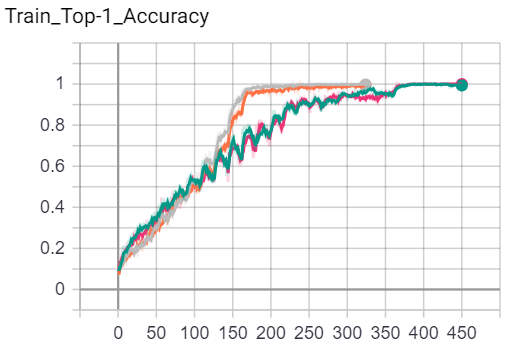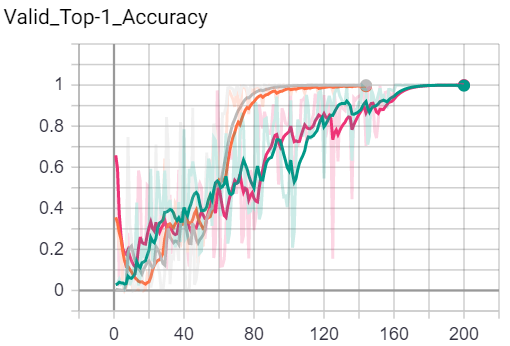## Short Conclusion

• OneCycle LR > Constant LR
• Making a new optimizer vs. Preserving `state` and re-using the same optimizer both achieve very similar performance. i.e. Discarding an optimizer’s `state` didn’t really hurt the model’s performance, with or without an LR Scheduler. Maybe the state is learned quickly.

Note: Conclusions are based on the Adam optimizer and OneCycle LR Scheduler. I haven’t experimented with other optimizers to see if dropping their `state` is more impactful

Edit Note: I’m not proposing to always throw optimizers away, I still believe the general guideline is to use the same optimizer and keep the history. Kindly share resources if anyone found results showing the importance of using the same optimizer :)